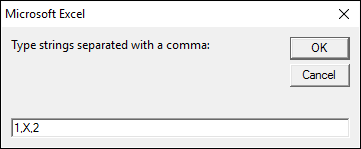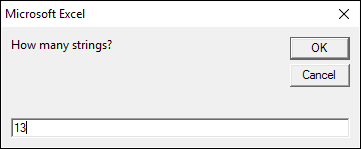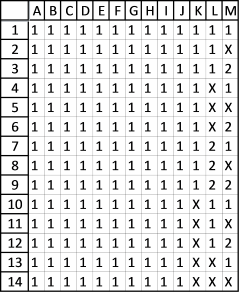Author: Oscar Cronquist Article last updated on February 13, 2018

Is there a way where i can predict all possible outcomes in excel in the below example.

Total games are 13 (ABCDEFGHIJKLM). Possible outcomes are win(home team),draw or win(Away team) represented by 1,X or 2 respectively.Outcomes should be in the below formats

111111111111X
11111111111XX
2222222X22221
222222222222X

The following macro lets you enter text strings separated by a comma and the number of games.

```Sub ListPermut()
'This macro creates a list of all permutations
'Get digital Help - http://www.get-digital-help.com/

'Define variables
Dim ws As Worksheet, Ans As String, ans1() As String, digits As Integer
Dim num As Integer, p As Long, i As Long, t As Long
Dim rng() As Long, c As Long, rng1() As String

'Insert a new sheet

Ans = InputBox("Type strings separated with a comma:")
digits = InputBox("How many strings?")

'Split text strings to an array
ans1 = Split(Ans, ",")

'Count values in aray
num = UBound(ans1) + 1

'Calculate number of permutations
p = num ^ digits

'Redimension arrays
ReDim rng(1 To digits)
ReDim rng1(1 To digits)

'Save 1 to all values in first row of array
For c = 1 To digits
rng(c) = 1
Next c

i = 0

'Don't show the result until finished
Application.ScreenUpdating = False

'Repeat until all permutations have been created
Do Until (i + t) = (p - 1)
'Use text strings instead of numbers
For c = LBound(rng1) To UBound(rng1)
rng1(c) = ans1(rng(c) - 1)
Next c
'Transfer values from array to worksheet
ws.Range("A1").Resize(, digits).Offset(i) = rng1

'Build next row of permutations
For c = digits To 1 Step -1
If c = digits Then
rng(c) = rng(c) + 1
ElseIf rng(c) = 0 Then
rng(c) = rng(c - 1)
End If
If rng(c) = num + 1 Then
rng(c) = 1
rng(c - 1) = rng(c - 1) + 1
End If
Next c

i = i + 1

'Insert a new sheet if rows exceed 999 999
If i = 1000000 Then
t = t + 1000000
i = 0
End If

Loop
'Use text strings instead of numbers
For c = LBound(rng1) To UBound(rng1)
rng1(c) = ans1(rng(c) - 1)
Next c
'Transfer values from array to worksheet
ws.Range("A1").Resize(, digits).Offset(i) = rng1
'Show output
Application.ScreenUpdating = True

End Sub
```

### Where to copy code?

1. Press Alt-F11 to open visual basic editor
2. Click Module on the Insert menu
3. Copy and paste code above to the code module4. Exit visual basic editor (Alt+Q)
5. Save your workbook as a *.xlsm file

### How to run macro?

1. Press Alt+F8 to open the macro dialog box
2. Click ListPermut macro
3. Click Run

### Instructions

Enter your text strings using a comma as a text delimiting character.Click OK button.

Enter number of games.Click OK button.

Excel processes the data for a while and then creates two sheets with a total of 1594323 permutations.The picture shows 14 out of 1594323 permutations.